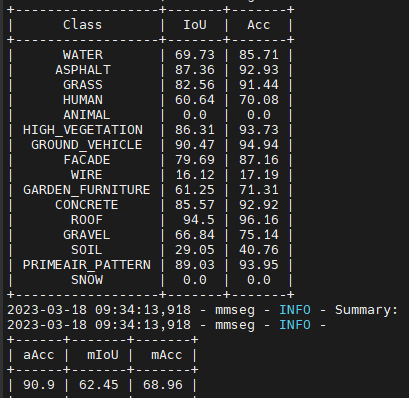# Train/validation split for the baseline model

Hi,

The dataset that the mmseg baseline model is trained on is split into train/validation according to this code:

``````+data = dict(
+    samples_per_gpu=2,
+    workers_per_gpu=2,
+    train=dict(
+        type=dataset_type,
+        data_root=data_root,
+        img_dir='inputs/train',
+        ann_dir='semantic_annotations/train',
+        pipeline=train_pipeline),
+    val=dict(
+        type=dataset_type,
+        data_root=data_root,
+        img_dir='inputs/val',
+        ann_dir='semantic_annotations/val',
+        pipeline=test_pipeline),
+    test=dict(
+        type=dataset_type,
+        data_root=data_root,
+        img_dir='inputs/val',
+        ann_dir='semantic_annotations/val',
+        pipeline=test_pipeline))
``````

However, as when using the dataset download link it is not split into two folders “train” and “val” for both the inputs and masks. I have manually put them into a 84/16 split. Midway through my model training, I noticed that class “ANIMAL” and “SNOW” does not show up in the validation set.Therefore, my questions are: how are they split in % and is it correct that the classes “ANIMAL” and “SNOW” are not present?

In order to find how the different categories are spread over the images, you will have to count the pixels per image.

Animal and snow are rare in this dataset, so 0.0 means that:

• either the animal and snow are not present in your validation set (I totally gave up on snow).
• or that the model hasn’t been able to recognize the animal and snow and is misclassifying these pixels in your current runs.